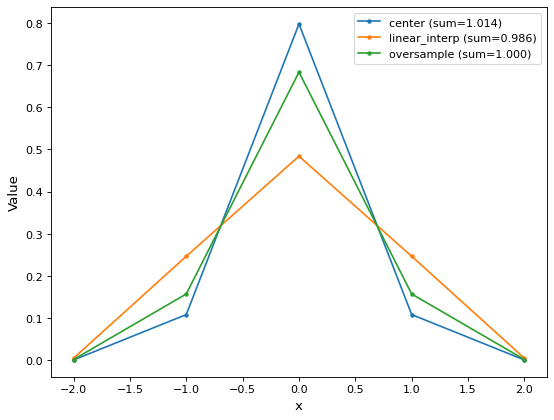# discretize_model¶

astropy.convolution.discretize_model(model, x_range, y_range=None, mode='center', factor=10)[source]

Function to evaluate analytical model functions on a grid.

So far the function can only deal with pixel coordinates.

Parameters
modelFittableModel or callable.

Analytic model function to be discretized. Callables, which are not an instances of FittableModel are passed to custom_model and then evaluated.

x_rangetuple

x range in which the model is evaluated. The difference between the upper an lower limit must be a whole number, so that the output array size is well defined.

y_rangetuple, optional

y range in which the model is evaluated. The difference between the upper an lower limit must be a whole number, so that the output array size is well defined. Necessary only for 2D models.

modestr, optional
One of the following modes:
• 'center' (default)

Discretize model by taking the value at the center of the bin.

• 'linear_interp'

Discretize model by linearly interpolating between the values at the corners of the bin. For 2D models interpolation is bilinear.

• 'oversample'

Discretize model by taking the average on an oversampled grid.

• 'integrate'

Discretize model by integrating the model over the bin using scipy.integrate.quad. Very slow.

factorfloat or int

Factor of oversampling. Default = 10.

Returns
arraynumpy.array

Model value array

Notes

The oversample mode allows to conserve the integral on a subpixel scale. Here is the example of a normalized Gaussian1D:

import matplotlib.pyplot as plt
import numpy as np
from astropy.modeling.models import Gaussian1D
from astropy.convolution.utils import discretize_model
gauss_1D = Gaussian1D(1 / (0.5 * np.sqrt(2 * np.pi)), 0, 0.5)
y_center = discretize_model(gauss_1D, (-2, 3), mode='center')
y_corner = discretize_model(gauss_1D, (-2, 3), mode='linear_interp')
y_oversample = discretize_model(gauss_1D, (-2, 3), mode='oversample')
plt.plot(y_center, label='center sum = {0:3f}'.format(y_center.sum()))
plt.plot(y_corner, label='linear_interp sum = {0:3f}'.format(y_corner.sum()))
plt.plot(y_oversample, label='oversample sum = {0:3f}'.format(y_oversample.sum()))
plt.xlabel('pixels')
plt.ylabel('value')
plt.legend()
plt.show()


(png, svg, pdf)